# Mathematics - Mathematical Analysis Total DifferentialSource

## Introduction

Hello it's a me again drifter1!

Last time I said that we would get into Double Integrals, but I forgot one more important thing that we will discuss today!

Today's topic of Mathematics are Total Differentials and how to check if an function f with this df exists.

There is not much more to say so let's get started!

## Linear approximation of a function

Suppose z = f(x, y) is a plane/surface and P0(x0, y0, z0) the point where the plane z and it's tangent plane cross each other.

We say that:

z = z0 + ∂f/∂x(P0) (x - x0) + ∂f/∂y (P0) (y - y0) = T(x, y)

where z0 = f(x0, y0).

In close points P(x, y) of P0(x0, y0) we have that: f(x, y) ~= T(x, y)

And so:

T(x, y) ~= f(x, y) = f(x0, y0) + fx(P0) (x - x0) + fy(P0) (y - y0)

is called the linear approximation of f(x, y) at P0.

## Total differential

This last thing takes us to something else.

Suppose that we now have f(x, y) = 0 as a plane and P0 (x0, y0).

We said that the derivative along a curve is:

df/dt =  ∂f/∂x * dx/dt + ∂f/∂y * dy/dt

This can also be re-written like that:

df/dt = fx(P0) * dx/dt + fy(P0) * dy/dt

If we now multiply with dt we get:

df= fx(P0) * dx + fy(P0) * dy

which is called the total differential of f at P0.

So, the derivative of a function f along a curve can then also be represented by a total differential.

But, can we also go in the other way?

For example:quicklatex

We want to check if an f(x, y) exists so that:

df = fx(P0)dx + fy(P0)dy

This means that: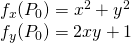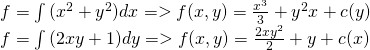Let's use the 2nd and 3rd equations to find c(y):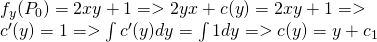So, then from the 3rd we have: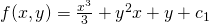We used this logic in Exact Differential equations, if you remember...

But, yes this worked this time, but will this work everytime?

## Checking if f exists with given df

If df = M(x, y)dx + N(x, y)dy = fxdx + fydx then we have:

fx = ∂f/∂x = M

and

fy = ∂f/∂y = N

A rule exists that says that the order of partial differentiation doesn't matter and so:

∂^2f/∂x∂y = ∂^2f/∂y∂x

This means that we can partially differentiate M and N:

∂^2f /∂y∂x = ∂M/∂y = My

∂^2f/∂x∂y = ∂N/∂x = Nx

My = Nx => ∂M/∂y = ∂N/∂x

So, a functions exists, when the condition My = Nx is true!

THIS is true for two variables!!!

By doing the same we can also find the conditions for 3 variables or more.

For 3 variables we have:

df = Mdx + Ndy + Pdz =>

My = Nx, Nz = Py and Mz = Px.

For example:

Let's check this condition for our previous example...which means that: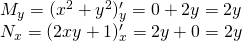And so:

My = 2y = Nx

which means that a function f exists with this total differential df.

And this is actually it for today and I hope that you enjoyed it!

Next time we will really get into Double integrals :P

Bye!

H2
H3
H4
3 columns
2 columns
1 column
Join the conversation now